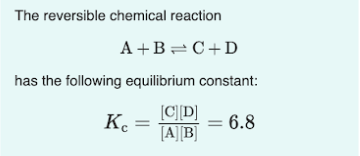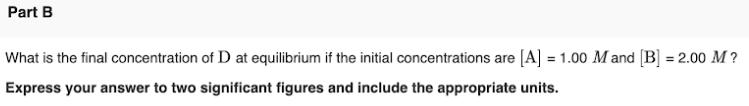# The reversible chemical reaction A + B ⇌ C + D has the equilibrium constant: Kc = [C][D]/[A][B] = 6.8 What is the final concentration of D at equilibrium if the initial concentrations are [A] = 1.00 M and [B] = 2.00 M? Express your answer to two significant figures and include the appropriate units.